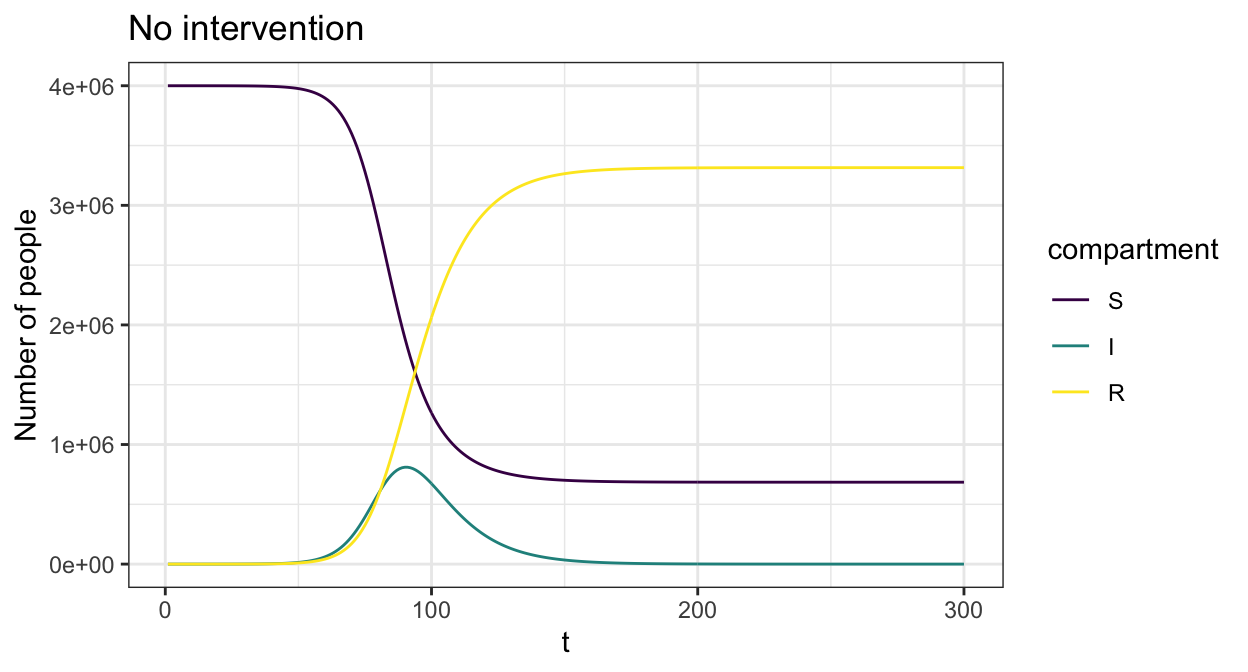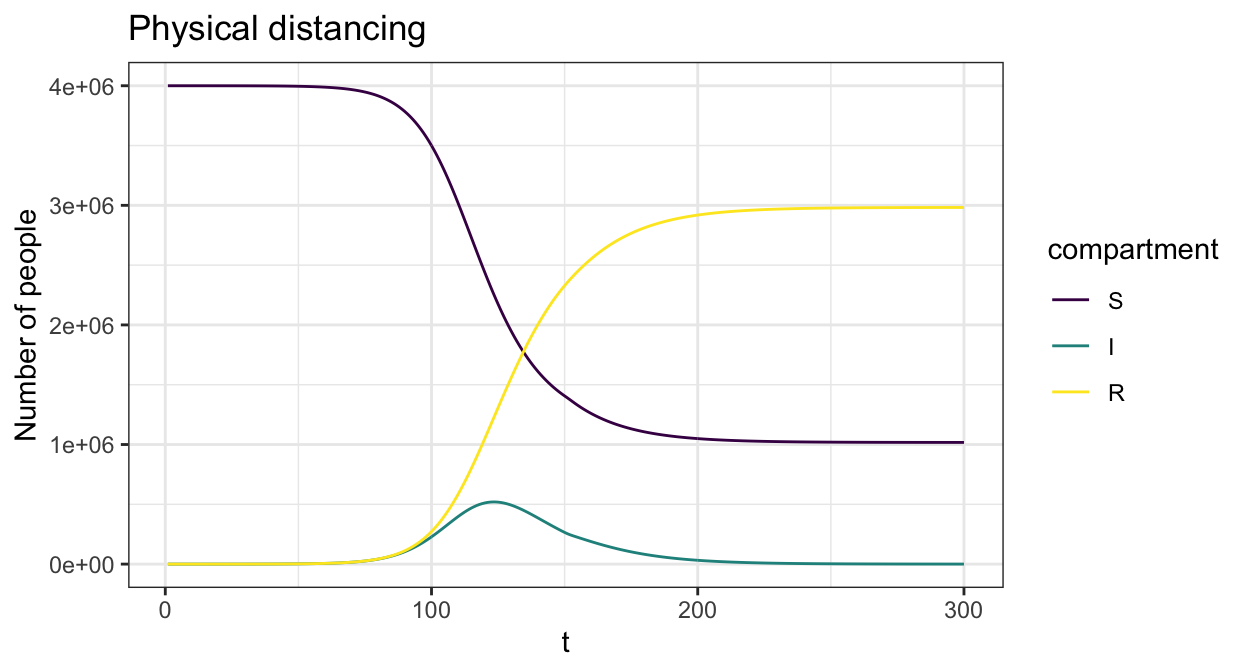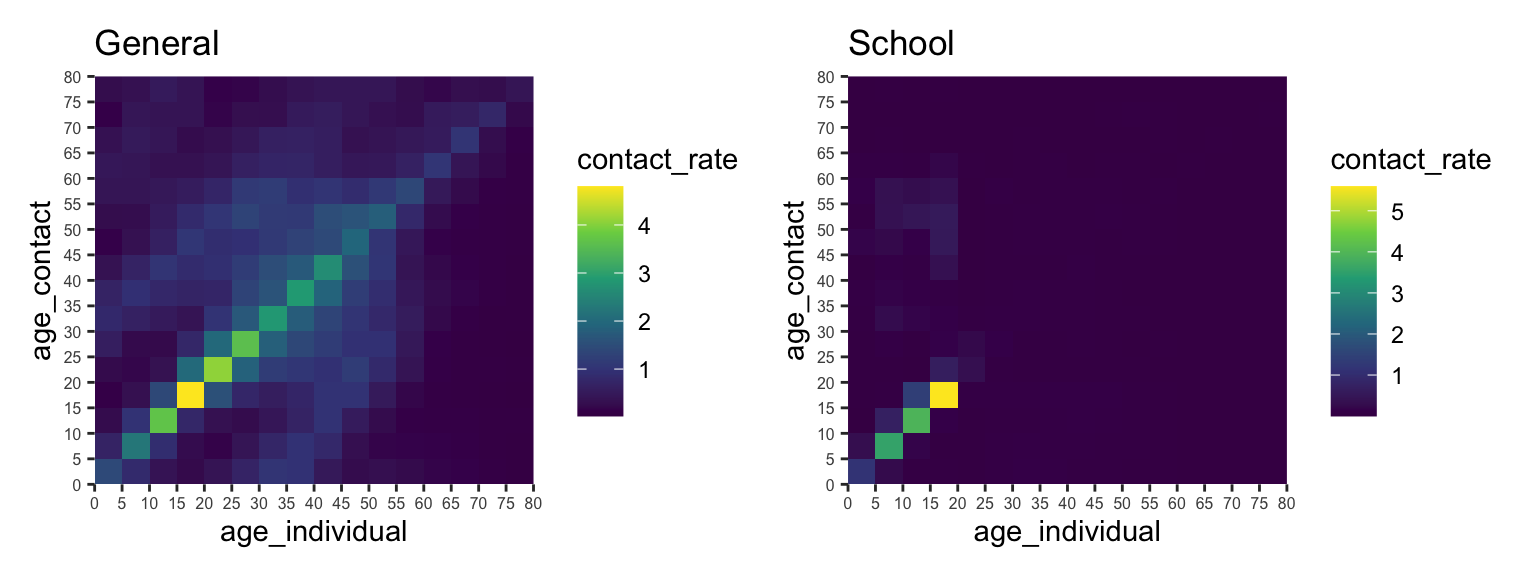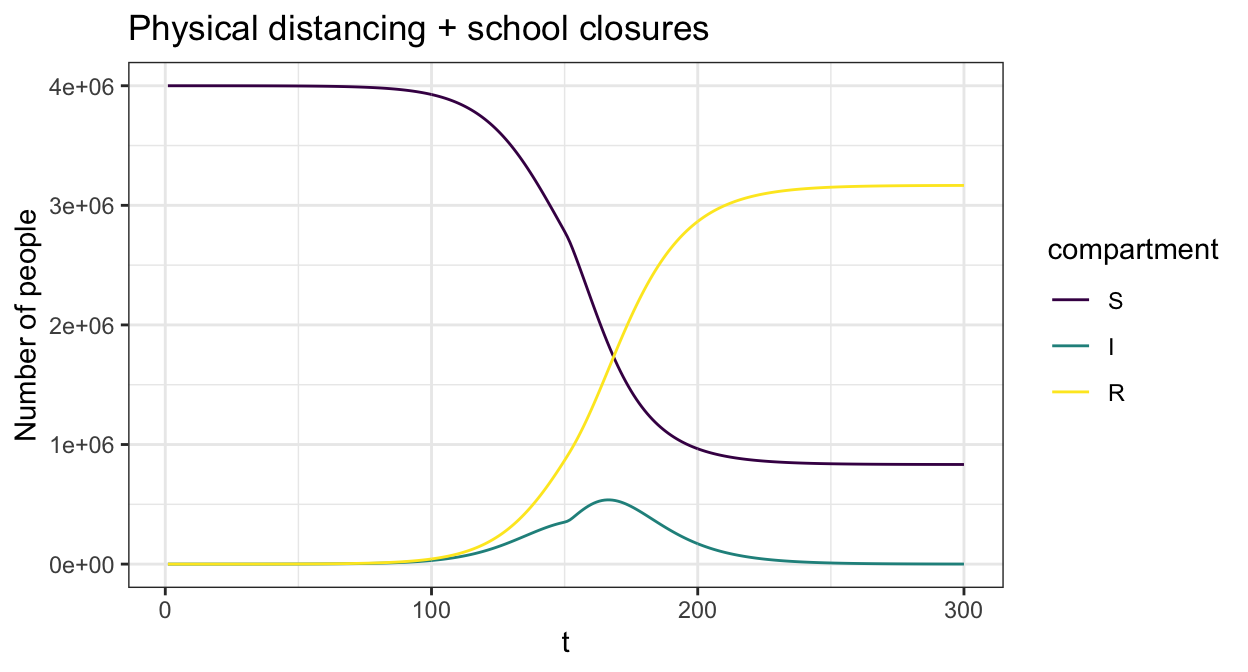# COVOID

The COVID-19 Open-source Infection Dynamics projectCOVOID is a R package for modelling COVID-19 and other infectious diseases using deterministic compartmental models (DCMs). It contains a built-in Shiny app enabling easy use and demonstration of key concepts to those without R programming backgrounds, along with an expanding API for simulating and estimating homogeneous and age-structured SIR, SEIR and extended models. In particular COVOID allows the simultaneous simulation of age specific (e.g. school closures) and general interventions over varying time intervals.

## Acknowledgements and support

Work on COVOID is facilitated by the generous assistance of Ian Sharp, philanthropic supporter of UNSW research.

If you would like to support our work, please see the UNSW Alumni & Giving web site for details of how to donate, or contact us directly at

## Installation

You can install the development version of COVOID from GitHub with:

``````
# install.packages("devtools")
devtools::install_github("cbdrh/covoid",build_vignettes = TRUE)``````

## An example

This is a basic introduction to COVOID package syntax. We demonstrate how to simulate an epidemic along with the impact of disease prevention policies using an age-structured SIR model. The populations demographics are matched to that of Australia, with a population of 4 million and 10 initial cases. The functions `import_contact_matrix` and `import_age_distribution` import data from Prem et al. (2017) (Prem, Cook, and Jit 2017) and the United Nations Population Division (2019) (United Nations Population Division 2019) on age specific contact rates and distributions, both grouped by 5 years. There are ~150 countries with both contact and age demographic information available in COVOID.

``````
library(covoid)
cm_oz <- import_contact_matrix("Australia","general")
p_age_oz <- import_age_distribution("Australia")
# initial conditions
S0 <- p_age_oz*4e6
I0 <- c(0,0,0,0,0,5,3,1,2,0,0,0,0,0,0,0)
R0 <- rep(0,length(S0))``````

We first consider the case without any interventions, in which the epidemic runs its course. The `sir_c_param` takes uses the basic reproduction number `R0` and the inverse of the average disease length `gamma` to parameterise the system of ODEs, and infer other parameters such the probability of disease transmission.

``````
param <- sir_c_param(R0 = 2.5,gamma = 0.1,cm=cm_oz,dist=p_age_oz)
state0 <- sir_c_state0(S0, I0, R0)
res <- simulate_sir_c(t = 300,state_t0 = state0,param = param)
plot(res,y=c("S","I","R"),main="No intervention")``````We can add in time varying interventions that reduce contact rates across the population using `create_contact_intervention()`. The below example reduces all physical contact by 20% in order to simulate physical distancing.

``````
phys_dist = contact_intervention(start = 10,stop = 150,reduce = 0.8,start_delay = 5,stop_delay = 5)
param <- sir_c_param(R0 = 2.5,gamma = 0.1,cm=cm_oz,dist=p_age_oz,contact_intervention = phys_dist)
state0 <- sir_c_state0(S0, I0, R0)
res <- simulate_sir_c(t = 300,state_t0 = state0,param = param)
plot(res,y=c("S","I","R"),main="Physical distancing")``````The COVOID package also allows you to separate the contact rates into different settings - e.g. school, work and home.

``````
cm_oz_all <- import_contact_matrix("Australia","general")
cm_oz_sch <- import_contact_matrix("Australia","school")
# separate out school and general population contact rates
cm_oz_all = cm_oz_all - cm_oz_sch
p_all <- plot(cm_oz_all) +
labs(title = "General") +
theme(axis.text.x = element_text(size=6, angle=0),
axis.text.y = element_text(size=6))
p_sch <- plot(cm_oz_sch) +
labs(title = "School") +
theme(axis.text.x = element_text(size=6, angle=0),
axis.text.y = element_text(size=6))

p_all + p_sch``````We can then add in time varying interventions using `create_intervention` to each setting. The below example reduces general contact by 20% to simulate physical distancing combined with a reduction in school age contact by 80% to simulate school closures.

``````
cm = list(all = cm_oz_all, sch = cm_oz_sch)
int = list(sch=contact_intervention(start = 10,stop = 150,reduce = 0.2,start_delay = 5,stop_delay = 5),
all=contact_intervention(start = 10,stop = 150,reduce = 0.8,start_delay = 5,stop_delay = 5))
param <- sir_c_param(R0 = 2.5,gamma = 0.1,cm=cm,dist=p_age_oz,contact_intervention = int)
state0 <- sir_c_state0(S0, I0, R0)
res <- simulate_sir_c(t = 300,state_t0 = state0,param = param)
plot(res,y=c("S","I","R"),main="Physical distancing + school closures")``````Prem, Kiesha, Alex R Cook, and Mark Jit. 2017. “Projecting Social Contact Matrices in 152 Countries Using Contact Surveys and Demographic Data.” PLoS Computational Biology 13 (9): e1005697.

United Nations Population Division. 2019. “World Population Prospects 2019, Online Edition.” United Nations, Department of Economic; Social Affairs.

### Corrections

If you see mistakes or want to suggest changes, please create an issue on the source repository.

### Reuse

Text and figures are licensed under Creative Commons Attribution CC BY 4.0. Source code is available at https://github.com/CBDRH/covoid, unless otherwise noted. The figures that have been reused from other sources don't fall under this license and can be recognized by a note in their caption: "Figure from ...".